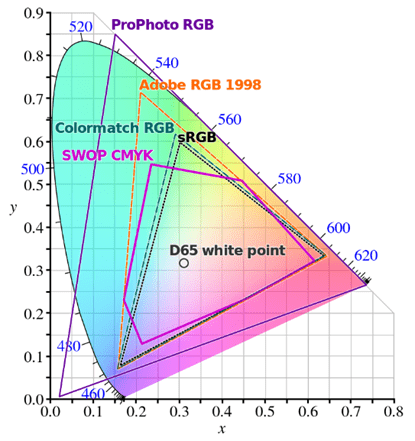# An introduction to colorimetry

Colorimetry is the discipline that measures the colour, employing numerical values to objectively represent them . In this post we will explain how we do the transition from human colour perception to an unambiguous way of defining colour.

The human retina possesses two types of light receptors: cone cells, which are responsible for day vision; and rod cells, which are in charge during the night. Cone cells have 3 peaks of sensitivity in short (“S”, 426 nm, associated with blue), medium (“M”, 530 nm, linked with green) and long (“L”, 560 nm, connected to red) wavelengths. This is why we employ trichromatic systems [2,3].

The human eye will not be able to distinguish 2 spectral distributions that stimulate the cones the same way, phenomenon that is called metamerism. As a result, a colour can be reproduced if we are able to create a spectral distribution that excites the cones as the original one .

CIE 1931 colour space was created in 1931 by the International Commission of Illumination (CIE, from the French name) in order to fulfil this purpose. It had been preceded by the publication in 1924 of the luminous efficiency function, V(λ), which shows the human eye’s  sensitivity to each wavelength for day light (see Figure 1). Therefore, 2 sources of light with the same radiance and different wavelength will seem to have different brightness due to our eyes’ perception .

CIE 1931 colour space was the result of combining the results of the research from William David Wright and John Guild. Their experiments consisted in asking a subject to combine 3 primary lights (blue at 435.8 nm, green at 546.1 nm and red at 700 nm) to produce the same colour as a reference monochromatic light. The results are shown in Figure 2, known as the 1931 RGB colour matching functions. These functions are scaled as if all the primary lights were equally bright, so in order to recover the absolute luminance for each light (R, G, B), this operation has to be reverted by dividing by a set of values provided with the functions .

The only fact that could be seen as strange in the plot is that there are negative values. The reason is that the 3 primary lights cannot be used to create every spectral colour. In these cases, some of the primary lights are mixed with the reference light (therefore a negative value is given to them) until a match is obtained between the new reference and the remaining primary lights .

However, it is difficult to visualize colours as a function of 3 parameters R, G, B. 3 new values r, g and b are obtained by dividing R, G, B by their total sum. Now, the sum of r, g and b is equal to 1, so it is enough with 2 variables (r and g, for instance) to define a colour, as b can be deduced from r, g ( b = 1 – r – g). By doing this, the rg chromaticity diagram can be plotted, see Figure 3. Be aware that colours with the same r, g, b have the same chromaticity but they could have different intensity (different R, G, B) .

The curve on the edge is called the spectral locus and each point corresponds to the chromaticity of a spectral colour (a monochromatic light). The points inside the curve correspond to non-spectral colours whereas the points outside the curve do not have any meaning. Furthermore, the triangle defined by the points R(1,0), G(0,1) and B(0,0) contains the colours that can be created with the RBG primaries, whereas the points inside the curve but outside the triangle are the ones created with other primary lights. For each primaries, the area inside the corresponding triangle is called gamut .

The CIE XYZ colour space is obtained from the 1931 XYZ colour matching functions, which are a linear transformation of the 1931 RGB colour matching functions. This was done so one of the functions (Y) matched very closely to the 1924 Luminosity Function, V(λ); and negative values could be avoided in the colour matching functions . The resulting colour matching functions and xy chromaticity diagram are the following ones:Figure 5. Colour gamuts of sRBG, Adobe RGB and CMYK, among other colour spaces, plotted on a CIE 1931 xy chromaticity diagram.

Let us see now the practical application of colour spaces. The most common colour spaces are the RGB colour space, used in computers or mobile phones; and the CMYK colour space, employed in printers.

The RGB model is an additive colour model in which red, green and blue are the primaries. However, they are not the same primaries as in the 1931 RGB colour space. The RGB model commonly refers to the sRGB colour space created by HP and Microsoft or the Adobe RGB colour space. The CMYK model is a subtractive colour model where cyan, magenta, yellow and key (black) are employed. Black is used instead of the combination of cyan, magenta and yellow to save ink and to obtain deeper black tones. The gamuts of these colour spaces, among others, can be seen in Figure 5.

Bibliography

1. Gilchrist, A.; Nobbs, J. Colorimetry, theory. In Encyclopedia of Spectroscopy and Spectrometry; Elsevier, 2016; pp. 328–333 ISBN 9780128032244.

2. Lucas, J.; Lucas, P.; Le Mercier, T.; Rollat, A.; Davenport, W. Applications of Rare Earth Luminescent Materials. In Rare Earths; Elsevier, 2015; pp. 281–318.

3. CIE 1931 color space – Wikipedia Available online: https://en.wikipedia.org/wiki/CIE_1931_color_space

4. A Beginner’s Guide to (CIE) Colorimetry | by Chandler Abraham | Color and Imaging | Medium Available online: https://medium.com/hipster-color-science/a-beginners-guide-to-colorimetry-401f1830b65a